Next: Example 3.3: Electric field Up: Electricity Previous: Example 3.1: Electrostatic force between

## Example 3.2: Electrostatic force between three non-colinear point charges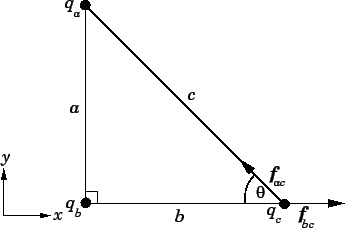Question: Suppose that three point charges,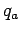,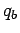, and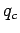, are arranged at the vertices of a right-angled triangle, as shown in the diagram. What is the magnitude and direction of the electrostatic force acting on the third charge if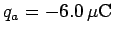,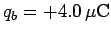,,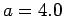m, and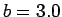m?

Solution: The magnitude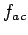of the force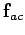exerted by chargeon chargeis given by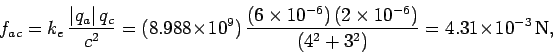where use has been made of the Pythagorean theorem. The force is attractive (since chargesandare of opposite sign). Hence, the force is directed from chargetowards charge, as shown in the diagram. The magnitude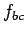of the force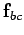exerted by chargeon chargeis given by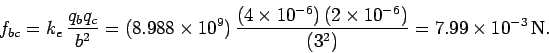The force is repulsive (since chargesandare of the same sign). Hence, the force is directed from chargetowards charge, as shown in the diagram. Now, the net force acting on chargeis the sum ofand. Unfortunately, since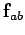andare vectors pointing in different directions, they cannot be added together algebraically. Fortunately, however, their components along the- and-axes can be added algebraically. Now, it is clear, from the diagram, thatis directed along the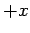-axis. If follows that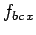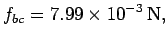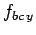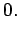It is also clear, from the diagram, thatsubtends an angle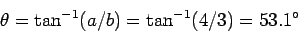with the-axis, and an angle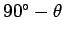with the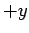-axis. It follows from the conventional laws of vector projection that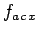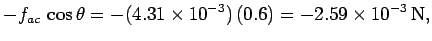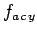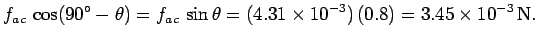The- and-components of the resultant force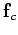acting on chargeare given by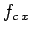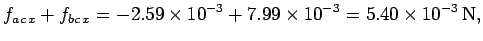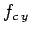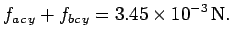Thus, from the Pythagorean theorem, the magnitude of the resultant force is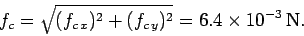Furthermore, the resultant force subtends an angle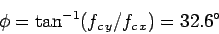with the-axis, and an angle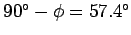with the-axis.Next: Example 3.3: Electric field Up: Electricity Previous: Example 3.1: Electrostatic force between
Richard Fitzpatrick 2007-07-14